一、填空

1．极限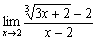=             .

2．设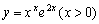，则y=             .

3．积分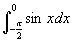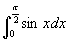的大小关系是             .

4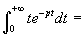.

5．设k是实常数，函数f (x)=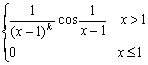.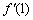存在，则k的取值范围是             .

1．设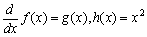，则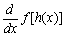等于：         .

Ag (x2)  B2xg (x)  Cx2g(x2)  D2xg (x2)

2．设f (x) x = x0处附近四阶连续可导且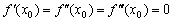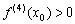，则有：

Ay = f (x)x = x0有极大值；    By = f (x)x = x0有极小值；

Cy = f (x)x = x0有拐点；      Dy = f (x)x = x0无极值也无拐点。

3．对于不定积分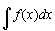，在下列等式中正确的是         .

A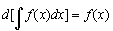B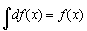C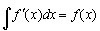D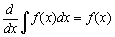.

4．已知f (x) x = 0的某邻域内连续，且f (0) =0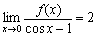，则在x=0处，f (x)         .

A）不可导  B）可导且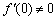C）取得极大值  D）取得极小值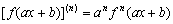2．求曲线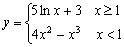的拐点坐标。

3．作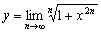的图形。

4．求曲线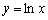上曲率最大的点的坐标。